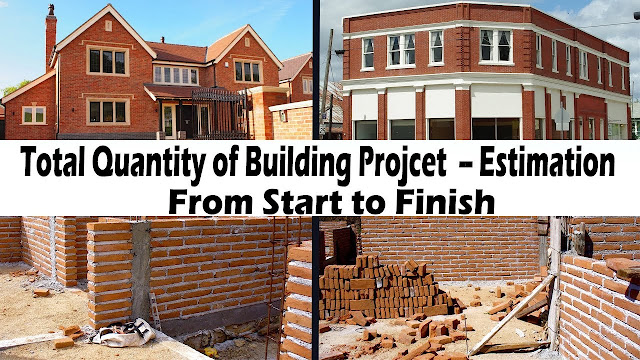How to calculate total quantity of building

# How to calculate total quantity of buildingIf you want to calculate the amount of various building materials required for construction before the project has started, the easiest method to do so is to refer the drawings. Now, depending on the type of structure to be built & the material quantity that you wish to calculate, the methodology will vary.
For example, in order to estimate the total amount of ceramic/vitrified tiles or marble quantity required in a residential building project, you first need to identify the areas where the tiles/marble will be used. In this case, the architectural drawings (prepared beforehand) will come in handy. The total amount of tiles or marble required for flooring, skirting, cladding, threshold, window sills & jambs, etc. for one typical floor can be estimated & multiplied with the total number of floors in the building. The same shall be estimated for the non-typical floors (refuge floors, fire-check floors, ground-floor, etc.) seperately.
The unit of measurement is another important factor which needs to be considered as for the same material, it may vary with it’s location of application. For example, the marble quantity required for flooring work can be estimated in sqm or sqft but the quantity of marble for the 75mm skirting work in staircase is conveniently measured in running meters (to simplify the calculation, as the width of skirting is predefined).

Similarly, the quantities of concrete, steel, brick, concrete blocks, glass, SS & MS railing, plaster, putty, pre-cast cement tiles etc. can also be calculated by referring to the project drawings & accepted specifications for the respective item of work. The quantities so calculated should also include the allowable wastage percentage of the respective building material.

In this Video Lecture I will disuse about Total quantity building step by step in detail.

Continue Reading about the "How to calculate total quantity of building" on the next page below